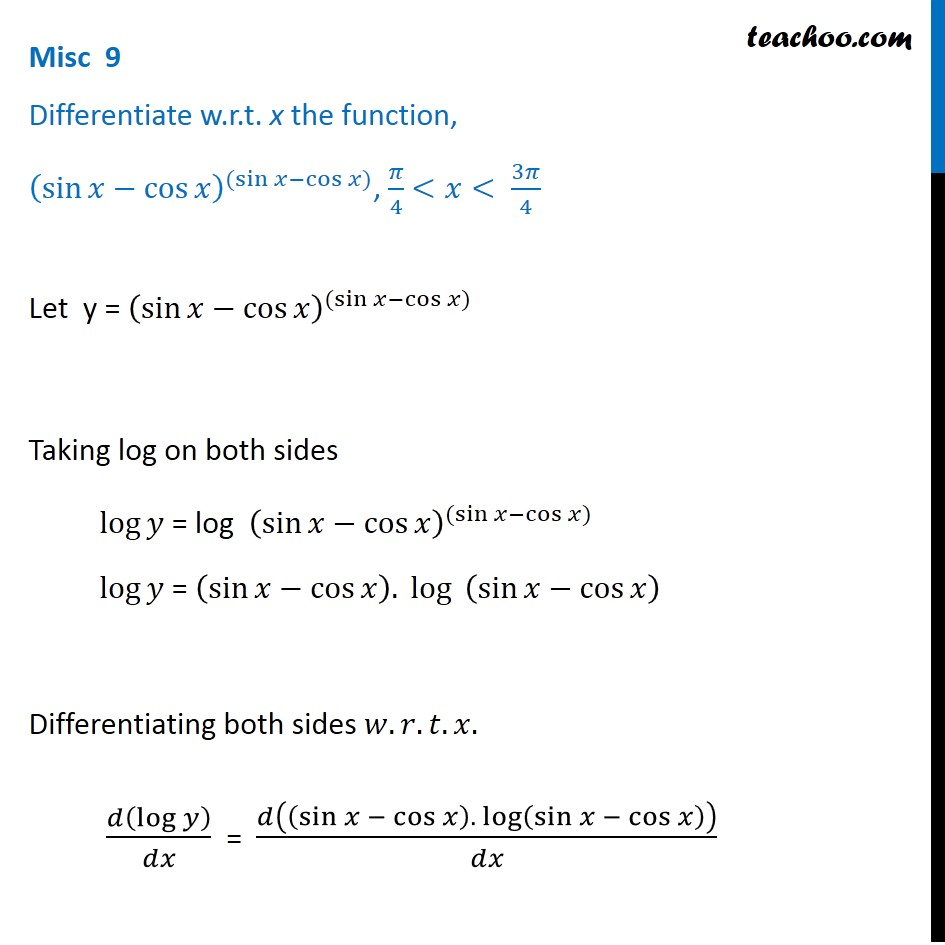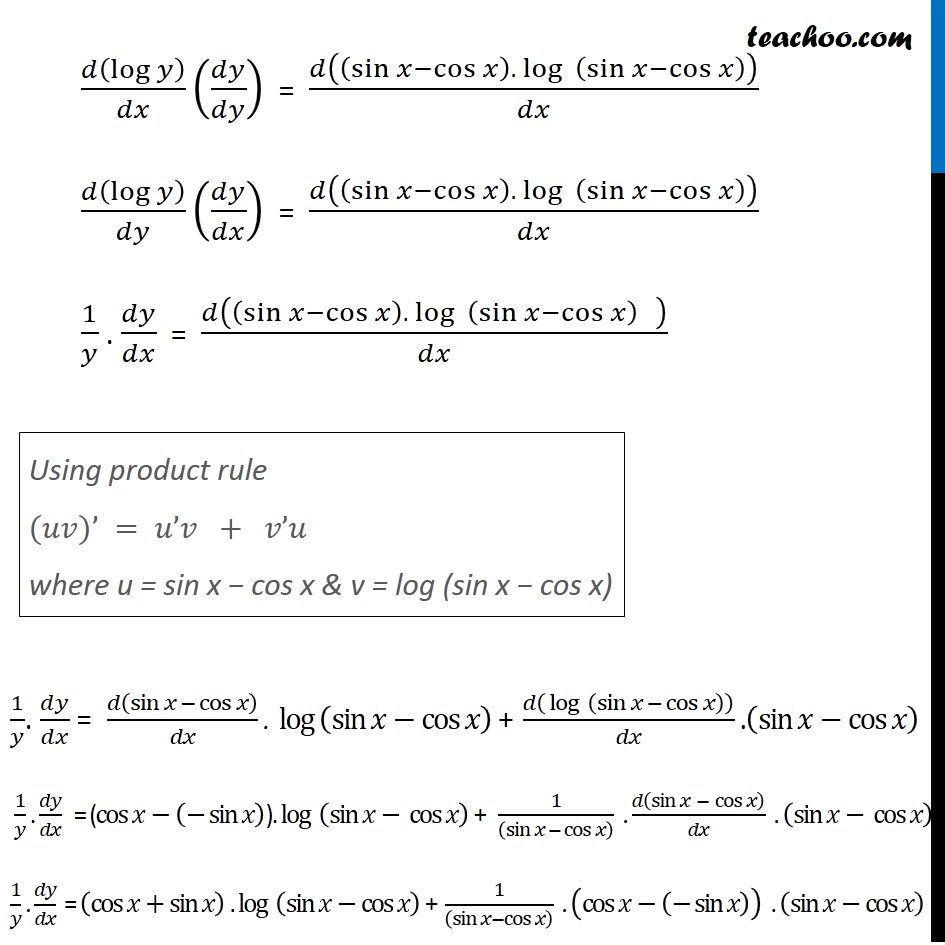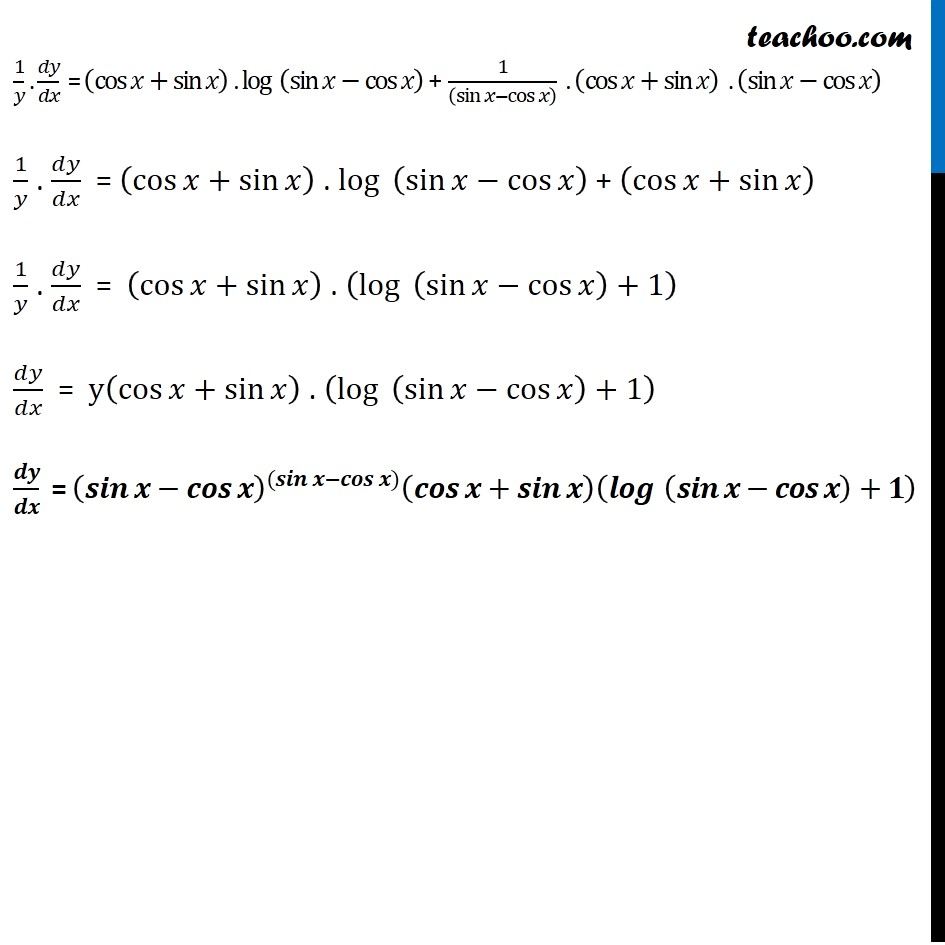Check Full Chapter Explained - Continuity and Differentiability - Continuity and Differentiability Class 121. Chapter 5 Class 12 Continuity and Differentiability
2. Serial order wise
3. Miscellaneous

Transcript

Misc 9 Differentiate w.r.t. x the function, (sin⁡𝑥−cos⁡𝑥 )^((sin⁡〖𝑥−cos⁡〖𝑥)〗 〗 ), 𝜋/4 <𝑥< 3𝜋/4 Let y = (sin⁡𝑥−cos⁡𝑥 )^((sin⁡〖𝑥−cos⁡〖𝑥)〗 〗 ) Taking log on both sides log⁡𝑦 = log (sin⁡𝑥−cos⁡𝑥 )^((sin⁡〖𝑥−cos⁡〖𝑥)〗 〗 ) log⁡𝑦 = (sin⁡𝑥−cos⁡𝑥 ). 〖 log〗⁡〖 (sin⁡𝑥−cos⁡𝑥 )〗 Differentiating both sides 𝑤.𝑟.𝑡.𝑥. 𝑑(log⁡𝑦 )/𝑑𝑥 = 𝑑((sin⁡𝑥 − cos⁡𝑥 ). 〖 log〗⁡(sin⁡𝑥 − cos⁡𝑥 ) )/𝑑𝑥 𝑑(log⁡𝑦 )/𝑑𝑥 (𝑑𝑦/𝑑𝑦) = 𝑑((sin⁡𝑥−cos⁡𝑥 ). 〖 log〗⁡〖 (sin⁡𝑥−cos⁡𝑥 )〗 )/𝑑𝑥 𝑑(log⁡𝑦 )/𝑑𝑦 (𝑑𝑦/𝑑𝑥) = 𝑑((sin⁡𝑥−cos⁡𝑥 ). 〖 log〗⁡〖 (sin⁡𝑥−cos⁡𝑥 )〗 )/𝑑𝑥 1/𝑦 . 𝑑𝑦/𝑑𝑥 = 𝑑((sin⁡𝑥−cos⁡𝑥 ). 〖 log〗⁡〖 (sin⁡𝑥−cos⁡𝑥 )〗 " " )/𝑑𝑥 " " 1/𝑦. 𝑑𝑦/𝑑𝑥 = 𝑑(sin⁡𝑥 − cos⁡𝑥 )/𝑑𝑥 . 〖 log 〗⁡(sin⁡𝑥−cos⁡𝑥 ) + 𝑑(〖 log〗⁡〖 (sin⁡𝑥 − cos⁡𝑥 )〗 )/𝑑𝑥 .(sin⁡𝑥−cos⁡𝑥 ) 1/𝑦 . 𝑑𝑦/𝑑𝑥 = (cos⁡𝑥−(−sin⁡𝑥 )). log⁡〖 (sin⁡𝑥−〖 cos〗⁡𝑥 )〗 + 1/((sin⁡𝑥 − cos⁡𝑥 ) ) . 𝑑(sin⁡𝑥 − cos⁡𝑥 )/𝑑𝑥 . (sin⁡𝑥−〖 cos〗⁡𝑥 ) 1/𝑦 . 𝑑𝑦/𝑑𝑥 = (cos⁡𝑥+sin⁡𝑥 ) . log⁡〖 (sin⁡𝑥−cos⁡𝑥 )〗 + 1/((sin⁡𝑥−cos⁡𝑥 ) ) . (cos⁡𝑥−(−sin⁡𝑥 )) . (sin⁡𝑥−cos⁡𝑥 ) Using product rule (𝑢𝑣)’ = 𝑢’𝑣 + 𝑣’𝑢 where u = sin x − cos x & v = log (sin x − cos x) 1/𝑦 . 𝑑𝑦/𝑑𝑥 = (cos⁡𝑥+sin⁡𝑥 ) . log⁡〖 (sin⁡𝑥−cos⁡𝑥 )〗 + 1/((sin⁡𝑥−cos⁡𝑥 ) ) . (cos⁡𝑥+sin⁡𝑥 ) . (sin⁡𝑥−cos⁡𝑥 ) 1/𝑦 . 𝑑𝑦/𝑑𝑥 = (cos⁡𝑥+sin⁡𝑥 ) . log⁡〖 (sin⁡𝑥−cos⁡𝑥 )〗 + (cos⁡𝑥+sin⁡𝑥 ) 1/𝑦 . 𝑑𝑦/𝑑𝑥 = (cos⁡𝑥+sin⁡𝑥 ) . (log⁡〖 (sin⁡𝑥−cos⁡𝑥 )+1〗 ) 𝑑𝑦/𝑑𝑥 = y(cos⁡𝑥+sin⁡𝑥 ) . (log⁡〖 (sin⁡𝑥−cos⁡𝑥 )+1〗 ) 𝒅𝒚/𝒅𝒙 = (𝒔𝒊𝒏⁡𝒙−𝒄𝒐𝒔⁡𝒙 )^((𝒔𝒊𝒏⁡〖𝒙−𝒄𝒐𝒔⁡〖𝒙)〗 〗 ) (𝒄𝒐𝒔⁡𝒙+𝒔𝒊𝒏⁡𝒙 )(𝒍𝒐𝒈⁡〖 (𝒔𝒊𝒏⁡𝒙−𝒄𝒐𝒔⁡𝒙 )+𝟏〗 )

Miscellaneous